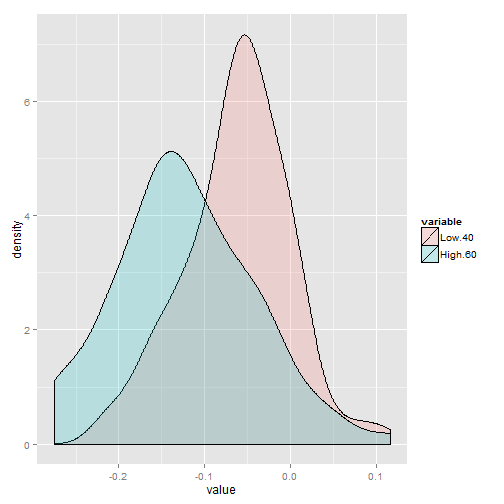# Anchoring financial advisor perception on performance

Anchoring is a well known and researched behavioral bias that affects individuals when trying to make an assessment or a prediction, for example, about an unknown future quantity or probability. Individuals begin from a starting reference to make a prediction, if the starting reference changes so does the prediction and this starting reference acts like an anchor curbing subsequent adjustments.

For example, if you ask a few individuals on a hot summer day “today, it feels pretty warm, what do you think the temperature is? I think it’s higher than 100° F (38° C)”. Unless all of them check it on a thermometer the answer you’re very likely to get changes as you change your starting reference of 100° changes: if you provide a higher ‘anchor’ you’ll get a higher average estimate and ceteris paribus if you supply a lower ‘anchor’.

During 180 training sessions held by me between January 2009 and June 2010 I collected a data sample of performance estimates provided by financial advisors who were asked to “assess reasonably your customer average performance during 2008: was it any different from a loss of 60%”. The following training session I asked the same question changing only the anchor, instead of a 60%-loss I used 40%-loss. A part from the fact these performances are extreme, the average result I got was expectedly, though little significant from a statistical point of view, what researchers have found out since the seventies (Kahneman and Tverski among the first ones).

Let’s pull off an informative plot of my data sample using R. The data contained in Anchoring.csv have been tweaked to demarcate the difference between the two anchors.

Let’s import the data in a data frame and have a look at the first 6 lines in it.

``````dat <- read.table(file = "Anchoring.csv", header = TRUE, sep = ";", dec = ",")
``````
``````##     Low.40  High.60
## 1 -0.06715       NA
## 2  0.01467       NA
## 3 -0.05461 -0.03835
## 4 -0.04103 -0.09547
## 5 -0.12915 -0.16800
## 6 -0.10425 -0.15439
``````

What we want is to plot the densities of these two distribution and compare them. Reshape2 provides the ‘melting’ function to adequately plot the distribution using ggplot2.

``````library(reshape2)
``````

We get the ‘molten’ data, and see what the data frame now looks like.

``````dat <- melt(dat, measured = 1:2)
``````
``````## Using as id variables
``````
``````head(dat)
``````
``````##   variable    value
## 1   Low.40 -0.06715
## 2   Low.40  0.01467
## 3   Low.40 -0.05461
## 4   Low.40 -0.04103
## 5   Low.40 -0.12915
## 6   Low.40 -0.10425
``````
``````tail(dat)
``````
``````##     variable    value
## 179  High.60 -0.21474
## 180  High.60 -0.13529
## 181  High.60 -0.10098
## 182  High.60 -0.23979
## 183  High.60 -0.03833
## 184  High.60 -0.14894
``````

Let’s load plyr and ggplot2 to perform respectively a simple summary statistic (the mean) and fot the actual plot.

``````library(ggplot2)
library(plyr)
``````

Summarize is an interesting summary function for our task at hand.

``````ddply(dat, .(variable), summarize, per_mean = mean(value, na.rm = TRUE))
``````
``````##   variable per_mean
## 1   Low.40 -0.06223
## 2  High.60 -0.12256
``````

The following plot shows how relevant anchoring can be…

``````qplot(data = dat, value, geom = "density", fill = variable, alpha = I(0.2))
``````
``````## Warning: Removed 2 rows containing non-finite values (stat_density).
``````As written before, these data have been tweaked to demarcate better the difference in the two distributions, though our little experiment points to the conclusions reached by others, I think it was an interesting confirmation of what we know about this powerful tool we, as individuals, unfortunately rely on extensively.

Posted in Economics, R-stats

### TweetsRodolfo Vanzini
@RodolfoVanzini